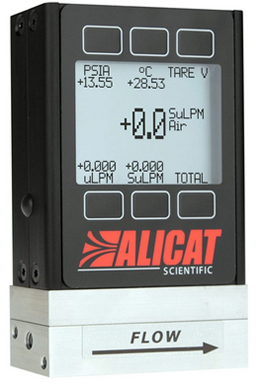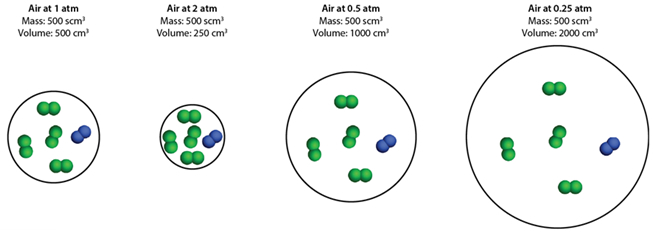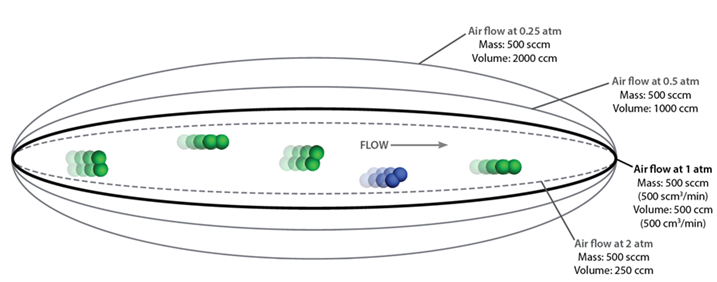## Mass vs Volumetric Flow: What is the Difference?

#### Simply put, one measures the number of molecules in a flowing gas, while the other measures the space occupied by said molecules.This article from Alicat explains the main difference between Mass & Volumetric Flow, and when we should use one or the other.

As it is commonly known, Mass flow measures the number of molecules in a flowing gas, whereas volumetric flow measures the space that those molecules occupy. As gases are compressible and widely affected by temperature, volumetric flow rates can significantly change depending on pressure and/or temperature changes.

Alicat present a simple yet accurate definition for both concepts, introducing the notions of actual flow rate & standardised (or normalised) volumetric flow rate. It reads as the following:

Volumetric flow rate is a measure of the 3-dimensional space that the gas occupies as it flows through the instrument under the measured pressure and temperature conditions. Volumetric flow rate can also be called actual flow rate.

Mass flow rate is a measure of the number of molecules that flow through the instrument, regardless of how much space those molecules occupy. Mass flow rate is often expressed as a standardised (or normalised) volumetric flow rate, which is the amount of space that those molecules would occupy if measured under standard temperature and pressure conditions (STP, or NTP).”

## Mass vs Volumetric: Which Measurement Do I Need to Use?

Most Alicat gas flow instruments - like the M-500SCCM-D Mass Flow Meter - can provide you with both mass & volumetric flow readings, and allow for closed loop control of either mass or volumetric flow. So, deciding which measurement the user needs for their specific application depends solely on the purpose of the application in question, what makes more sense regarding what the user is trying to achieve.

To get a better idea of how one can make that decision, Alicat introduce a connection with “The Ideal Gas Law”, which describes the relationship between mass, volume, pressure and temperature for static gases: PV=nRT

P = Static pressure | V = Volume | n = Molar mass | R = Gas constant | T = Absolute temperature

This means that if the gas pressure (P) increases by a factor of 2, the volume of the gas (V) decreases by a factor of 2 – if all other factors are constant. This happens because gases are compressible, and their molecules are pressed closer to each other as pressure increases.

If the pressure decreases by half, volume doubles. The molar mass (n, the number of particles in the volume) stays the same, regardless of changes in static pressure.#### To help explain this, Alicat have come up with an example:

Imagine a flexible container filled with 500 cm3 of air at standard ambient temperature (25 °C) and at atmospheric pressure (1 atm, about 14.696 psia). By defining these conditions as standard (STP), then the mass can be expressed as a standardised volume (scm3).

Compressing the volume to 250 cm3, will make the air molecules move closer together, increasing the pressure to 2 atm. Now, pressure will increase but the molar mass (the number of molecules) is still the same as in the 500 scm3 volume.

If the volume is increased to 1000 cm3, the original pressure of 1 atm is halved to 0.5 atm. Again, if we double the volume to 2000 cm3 the pressure will once again be reduced to half (0.25 atm) – but the mass remains the same.

In each scenario, the mass of air inside the container never changes, because no air has been removed or added, only the STP has changed.

### Flow Rates and The Ideal Gas Law

When air is put into motion as a flow of gas, the actual space that the air takes up per unit of time (volumetric flow rate) varies with pressure in the same manner as the static air.

Doubling the line pressure halves the volumetric flow rate, and vice versa. However, the number of molecules of air that flow per unit of time (mass flow rate) does not change.## Mass vs Volumetric: Proof is in the Purpose!

Ultimately, the decision between one or the other, has to lay on the purpose and focus behind the application.

Mass Flow: when the focus is the gas itself, and the number of molecules needs to be monitored, disregarding pressure conditions, such as Gas Mixing and Custody Transfer applications.

• Gas mixing applications: to ensure that the concentrations of the constituent gases remain constant relative to each other
• Custody transfer applications: where the mass of the gas being used is more critical than the space that mass fills.

Volumetric Flow: if the focus is what is in the gas volume, like monitoring the components of a gas stream under actual process conditions.

Applications such as Industrial Hygiene and Ambient Air Monitoring benefit more from volumetric flow as the objective is usually to quantify the number of certain particles within the volume of air, under the actual measured conditions.

Should you like to know more about Mass & Volumetric Flow, please contact our Sales Team. We will be happy to find you the right instrument to fit your application requirements. You can also see the Mass Flow Meter ranges we have available on our website.